NCERT Maths Solutions for Ex 2.2 class 10 Polynomials is the perfect guide to boost up your preparation during CBSE 10th Class Maths Examination.

## RS Aggarwal Class 10 Solutions Chapter 2 Polynomials Ex 2B

These Solutions are part of RS Aggarwal Solutions Class 10. Here we have given RS Aggarwal Class 10 Solutions Chapter 2 Polynomials Ex 2B. You must go through NCERT Solutions for Class 10 Maths to get better score in CBSE Board exams along with RS Aggarwal Class 10 Solutions.

Question 1.
Solution:
Zeros are 3, -2, 1 of
p(x) = x3 – 2x2 – 5x + 6
Here, a = 1, b = -2, c = -5, d = 6
We know that if α, β and γ are the roots of
f(x) = ax3 + bx2 + cx + d, thenQuestion 2.
Solution:
Zeros are 5, -2 and $$\frac { 1 }{ 3 }$$ ofQuestion 3.
Solution: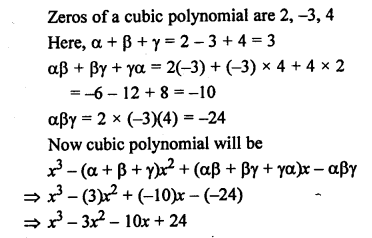Question 4.
Solution: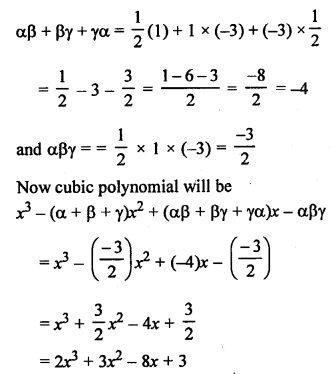Question 5.
Solution: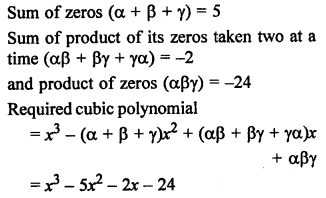Find the quotient and the remainder when:
Question 6.
Solution: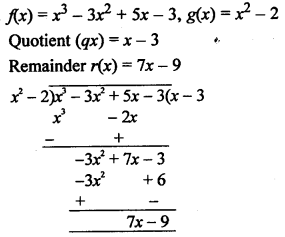Question 7.
Solution: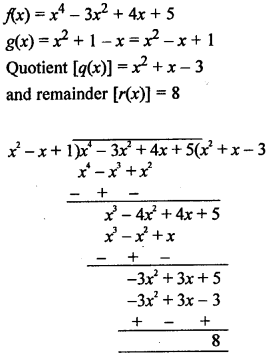Question 8.
Solution:Question 9.
Solution:
f(x) = 2x4 + 3x3 – 2x2 – 9x – 12
g(x) = x2 – 3
Quotient [q(x)] = 2x2 + 3x + 4
Remainder [r(x)] = 0
Remainder is zero.
x2 – 3 is a factor of f(x)Question 10.
Solution: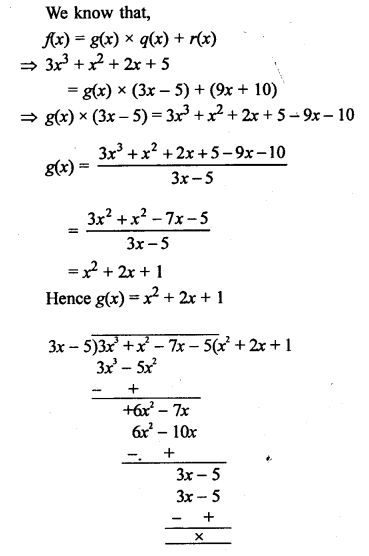Question 11.
Solution: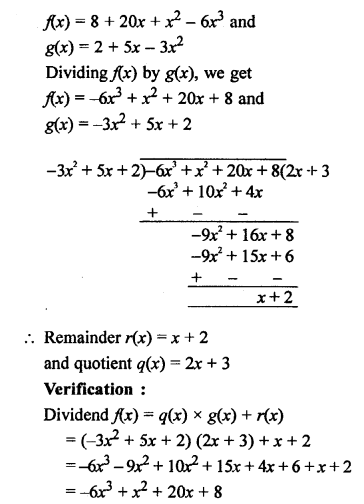Question 12.
Solution:= (x + 1) (x + 4) (x – 3)
If x + 4 = 0, then x = -4
If x – 3 = 0, then x = 3
Zeros are -1, -4, 3

Question 13.
Solution: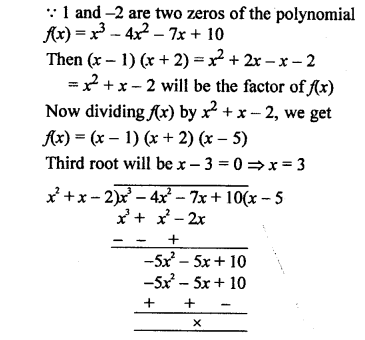Question 14.
Solution: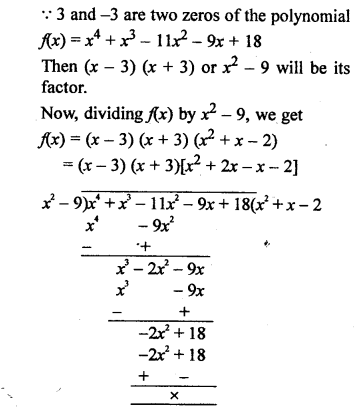=> (x – 3) (x + 3) [x (x + 2) – 1 (x + 2)]
=> (x – 3) (x + 3) (x + 2) (x – 1)
Other zeros will be
If x + 2 = 0 then x = -2
and if x – 1 =0, then x = 1
Zeros are 3, -3, -2, 1

Question 15.
Solution:
2 and -2 are the two zeros of the polynomial
f(x) = x4 + x3 – 34x2 – 4x + 120,
Then (x – 2) (x + 2) or x2 – 4 will its the factor of f(x)
Now dividing f(x) by x2 – 4, we get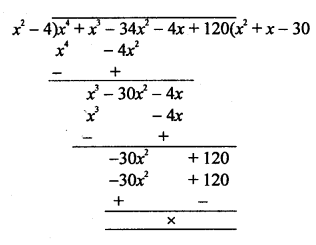f(x) = (x – 2) (x + 2) (x2 + x – 30)
= (x – 2)(x + 2)[x2 + 6x – 5x – 30]
= (x – 2) (x + 2)[x(x + 6) – 5(x + 6)]
= (x – 2) (x + 2) (x + 6) (x – 5)
Other two zeros are
If x + 6 = 0, then x = -6 and
if x – 5 = 0, then x = 5
Roots of f(x) are 2, -2, -6, 5

Question 16.
Solution: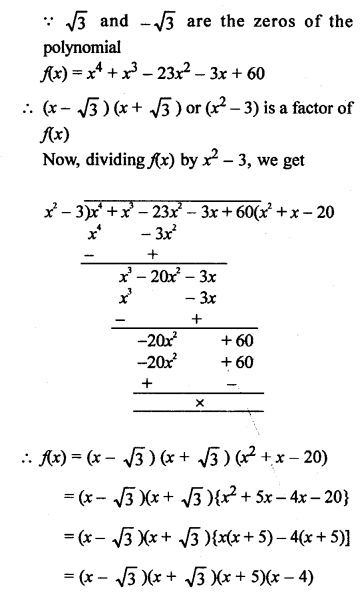Other two zeros are :
if x + 5 = 0, then x = -5
and if x – 4 = 0, then x = 4
Hence, all the zeros of f(x) are : √3, – √3, 4, -5

Question 17.
Solution:
√3 and – √3 are the zeros of the polynomial
f(x) = 2x4 – 3x3 – 5x2 + 9x – 3
=> (x – √3) (x + √3) or (x2 – 3) is a factor of f(x)
Now, dividing f(x) by x2 – 3, we getQuestion 18.
Solution: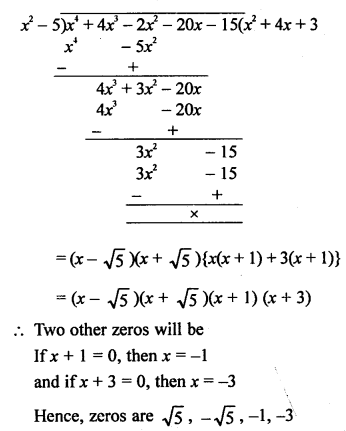Question 19.
Solution:Hope given RS Aggarwal Solutions Class 10 Chapter 2 Polynomials Ex 2B are helpful to complete your math homework.

If you have any doubts, please comment below. Learn Insta try to provide online math tutoring for you.